# 1 Kirsten Wickelgren, Talk 1 (Wednesday, July 14)

## 1.1 Intro

Abstract:

Morel and Voevodsky’s $${\mathbb{A}}^1$$ homotopy theory imports tools from algebraic topology into the study of schemes, or in other words, into the study of the solutions to polynomial equations. This theory produces greater understanding of arithmetic and geometric aspects these solutions. We will introduce some of this theory using as a guide questions such as “How many lines meet 4 lines in 3-space?”

References/Background:

Enumerative geometry counts algebro-geometric objects over $${\mathbb{C}}$$. Example: how many lines meet 4 generic lines in $${\mathbb{P}}^3$$? The answer is 2, and our goal is to record this kind of arithmetic information about geometric objects over a field $$k$$ whose intersections are fixed over $$\mkern 1.5mu\overline{\mkern-1.5muk\mkern-1.5mu}\mkern 1.5mu$$ but not necessarily $$k$$ itself. Our main tool will be $${\mathbb{A}}^1{\hbox{-}}$$homotopy theory, due to Morel-Voevodsky.

## 1.2 Classical Theory

First some classical homotopy theory. The sphere can be defined as \begin{align*} S^n \mathrel{\vcenter{:}}=\left\{{{ {x}_1, {x}_2, \cdots, {x}_{n}} {~\mathrel{\Big|}~}\sum x_i^2 = 1}\right\} \simeq{\mathbb{P}}^n({\mathbb{R}}) / {\mathbb{P}}^{n-1}({\mathbb{R}}) ,\end{align*} and we have a degree map $$[S^n, S^n] \to {\mathbb{Z}}$$. Given any $$f\in {\mathsf{Top}}(S^n, S^n)$$ and $$p\in S^n$$, we can write $$f^{-1}(p) = \left\{{{ {q}_1, {q}_2, \cdots, {q}_{N}}}\right\}$$ and compute $$\deg f = \sum_{i=1}^N \deg_{q_i} f$$ in terms of local degrees. Letting $$V$$ be a ball containing $$p$$, we have $$F^{-1}(V) \supseteq U \ni q_i$$ another ball such that $$U \cap f^{-1}(p) = q_i$$. Then $$U/{{\partial}}U \simeq S^n \simeq V/{{\partial}}V$$, so we can define a map \begin{align*} \mkern 1.5mu\overline{\mkern-1.5muf\mkern-1.5mu}\mkern 1.5mu: {U \over U\setminus\left\{{q_i}\right\}} \to {V \over V\setminus\left\{{p}\right\}} \end{align*} and define $$\deg_{q_i} f \mathrel{\vcenter{:}}=\deg \mkern 1.5mu\overline{\mkern-1.5muf\mkern-1.5mu}\mkern 1.5mu$$.

Letting $${\left[ {{ {x}_1, {x}_2, \cdots, {x}_{n}}} \right]}$$ be oriented coordinates about $$q_i$$ and $${\left[ {{ {y}_1, {y}_2, \cdots, {y}_{n}}} \right]}$$ about $$p$$, then $$f = {\left[ {{ {f}_1, {f}_2, \cdots, {f}_{n}}} \right]}: {\mathbb{R}}^n\to {\mathbb{R}}^n$$ and we can consider $$J_f \mathrel{\vcenter{:}}=\operatorname{det}\qty{{\partial}f_i \over {\partial}x_i}$$. There is then a formula \begin{align*} \deg_{q_i}(f) = \begin{cases} +1 & J_f(q_i) > 0 \\ -1 & J_f(q_i) < 0. \end{cases} ,\end{align*} and for all $$q_i$$ we have $$\deg f = \# f^{-1}({\operatorname{pt}})$$, i.e. the number of solutions to the polynomial system $$\left\{{f_1 = f_2 = \cdots f_n = 0}\right\}$$.

If $$f\in {\mathbb{C}}[x]$$ of degree $$n$$, we can regard $$f$$ as a function $$f: {\mathbb{P}}^1({\mathbb{C}})\to {\mathbb{P}}^1({\mathbb{C}})$$ and by the fundamental theorem of algebra, \begin{align*} \deg f = n = \# \left\{{f=0}\right\} .\end{align*}

We can similarly count solutions to $$f=0$$ when $$f$$ is a section of a rank $$n$$ vector bundle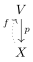This count can be computed using the Euler class: \begin{align*} e(V) = e(V, f) = \sum_{q_i\in \left\{{f=0}\right\}} \deg q_i f .\end{align*}

Let $$X \mathrel{\vcenter{:}}={\operatorname{Gr}}(1, 3)_{/{\mathbb{C}}}$$, the Grassmannian parameterizing dimension 2 subspaces $$W \subseteq {\mathbb{C}}^4$$, or equivalently lines in $${\mathbb{P}}W \subseteq {\mathbb{P}}({\mathbb{C}}^4) \cong {\mathbb{P}}^3({\mathbb{C}})$$, where $${\mathbb{P}}W$$ is defined as $$W\setminus\left\{{0}\right\}$$ where $$\lambda w\sim w$$ for any $$\lambda \in {\mathbb{C}}^{\times}$$. The tautological is a rank 2 bundle:Let $$L_1, \cdots, L_4$$ be four lines in $${\mathbb{P}}^3$$, then $$\left\{{\text{lines intersecting all } L_i}\right\} = \left\{{f=0}\right\}$$ where $$f$$ is a section (depending on the $$L_i$$) of the bundleand the Euler number of this bundle counts the number of such intersections. In particular, $$e({\mathcal{E}})$$ is independent of the choice of lines and section, provided they’re sufficiently generic (so the $$L_i$$ do not pairwise intersect). Using the splitting principle and knowledge of $$H^*({\operatorname{Gr}})$$, one can compute $$e({\mathcal{E}}) = 2$$.

## 1.3 Over arbitrary fields: Grothendieck-Witt

We’d like to do this over arbitrary fields $$k$$. Lannes and Morel defined degrees for rational maps $$f:{\mathbb{P}}^1 \to {\mathbb{P}}^1$$. Above we only remembered the sign of $$J_f$$, and here we’ll allow remembering more: $$\deg f$$ will be valued in $${\operatorname{GW}}(k)$$. We can realize $${\operatorname{GW}}(k)$$ as the group completion of the semiring of nondegenerate symmetric bilinear forms under $$\perp, \otimes_k$$, where we complete with respect to $$\perp$$. It is related to the Witt group by \begin{align*} W(K) { { \, \xrightarrow{\sim}\, }}{{\operatorname{GW}}(k) \over {\mathbb{Z}}\left\langle{q_{ {\operatorname{hyp}}} }\right\rangle } \mathrel{\vcenter{:}}={{\operatorname{GW}}(k) \over {\mathbb{Z}} { \left[ {\left\langle{1}\right\rangle + \left\langle{-1}\right\rangle} \right] } } .\end{align*} There is a rank map \begin{align*} \operatorname{rank}: {\operatorname{GW}}(k) &\to {\mathbb{Z}}\\ q: (V{ {}^{ \scriptstyle\otimes_{k}^{2} } } \to K) &\mapsto \dim_k V ,\end{align*} which can be realized by a pullbackWe can also write $${\operatorname{GW}}(k)$$ in terms of generators $$\left\langle{a}\right\rangle$$ where $$a\in k^{\times}/ (k^{\times})^{\times 2}$$, where $$\left\langle{a}\right\rangle$$ is associated to a bilinear form \begin{align*} \left\langle{a}\right\rangle: k^{\times 2} &\to k \\ (x, y) &\mapsto axy ,\end{align*} subject to relations

• $$\left\langle{a}\right\rangle\left\langle{b}\right\rangle = \left\langle{ab}\right\rangle$$
• $$\left\langle{u}\right\rangle + \left\langle{v}\right\rangle = \left\langle{uv(u+v)}\right\rangle + \left\langle{u+v}\right\rangle$$
• $$\left\langle{u}\right\rangle + \left\langle{-u}\right\rangle = \left\langle{1}\right\rangle + \left\langle{-1}\right\rangle = q_{\operatorname{hyp}}$$, which is the matrix \begin{align*} q_{\operatorname{hyp}}= { \begin{bmatrix} {0} & {1} \\ {1} & {0} \end{bmatrix} } .\end{align*}

The signature is the difference between the numbers of positive and negative ones in the associated matrix, and one can show \begin{align*} \operatorname{rank}: {\operatorname{GW}}({\mathbb{C}}) &{ { \, \xrightarrow{\sim}\, }}{\mathbb{Z}}\\ (\operatorname{rank}, \operatorname{sig}): {\operatorname{GW}}({\mathbb{R}}) &{ { \, \xrightarrow{\sim}\, }}{\mathbb{Z}}^{\times 2} \\ (\operatorname{rank}, {\operatorname{disc}}): {\operatorname{GW}}({\mathbb{F}}_q) &{ { \, \xrightarrow{\sim}\, }}{\mathbb{Z}}\times{\mathbb{F}}_q^{\times}/ ({\mathbb{F}}_q^{\times})^{\times 2} \end{align*} where the last is a situation where we can compute étale cohomology.

Let $$k\in \mathsf{Field}$$ be complete and discretely valued with residue field $$\kappa$$, e.g. $$k= { {\mathbb{Q}}_p }$$ or $${\mathbb{F}}_p{\left(\left( t \right)\right) }$$ with $$\kappa = {\mathbb{F}}_p$$. Then if $$\operatorname{ch}k \neq 2$$, \begin{align*} GW(k) { { \, \xrightarrow{\sim}\, }}W(k){ {}^{ \scriptscriptstyle\oplus^{2} } } .\end{align*}

For $$E_{/ {k}}$$ a finite separable field extension, we’ll have transfers \begin{align*} \operatorname{Tr}_{E_{/ {k}} }: {\operatorname{GW}}(E) &\to {\operatorname{GW}}(k) \\ (V{ {}^{ \scriptstyle\otimes_{k}^{2} } } \xrightarrow{\beta} E) &\mapsto (V{ {}^{ \scriptstyle\otimes_{k}^{2} } } \xrightarrow{\beta} E \xrightarrow{\operatorname{Tr}_{E_{/k}}} k) ,\end{align*} which coincide with classical transfers for field extensions.

For Lannes/Morel’s formula, given $${\mathbb{P}}^1_{/ {k}} \xrightarrow{f} {\mathbb{P}}^1_{/ {k}}$$ and $$p\in {\mathbb{P}}^1_{/ {k}}$$, we can write $$f^{-1}(p) = \left\{{{ {q}_1, {q}_2, \cdots, {q}_{N}}}\right\}$$ and suppose $$J(q_i) = f'(q_i) \neq 0$$ for all $$i$$. Then we remember the entire Jacobian and set \begin{align*} \deg f \mathrel{\vcenter{:}}=\sum_{i=1}^N \operatorname{Tr}_{k(q_i)_{/ {k}} } \left\langle{J(q_i)}\right\rangle ,\end{align*} which in fact doesn’t depend on $$p$$. Morel defines an $${\mathbb{A}}^1{\hbox{-}}$$degree \begin{align*} \deg^{{\mathbb{A}}^1}: [{\mathbb{P}}^n/{\mathbb{P}}^{n+1}, {\mathbb{P}}^n/{\mathbb{P}}^{n+1}]^{{\mathbb{A}}^1} \to {\operatorname{GW}}(k) ,\end{align*} where we are taking unstable $${\mathbb{A}}^1{\hbox{-}}$$homotopy classes of maps. Noting that an element of $${\operatorname{GW}}({\mathbb{R}})$$ was determined by its rank and signature, we get a commutative diagram showing that $$\deg^{{\mathbb{A}}^1}$$ is compatible with rank, signature, and the classical algebraic topological degree. There are other ways of computing this degree besides taking the above sum: Cazanave, Brazelton-McKean-Pauli give formulas in terms of Bézoutians.

## 1.4 Homotopy

Recall that \begin{align*} X\wedge Y \mathrel{\vcenter{:}}={ X\times Y \over (X\times{\operatorname{pt}}) \cup({\operatorname{pt}}\times Y)} && \in {\mathsf{Top}}_* ,\end{align*} and $$S^n \wedge S^m \xrightarrow{\sim} S^{n+m}$$ and $$(S^1){ {}^{ \scriptscriptstyle\wedge^{n} } } \xrightarrow{\sim} S^n$$, so we define \begin{align*} {\Sigma}_{S^1} X \mathrel{\vcenter{:}}= S^1 \wedge X .\end{align*} In $${\mathbb{A}}^1$$ homotopy theory we declare $${\mathbb{A}}^1 \simeq{\operatorname{pt}}$$.

We can take a pushout of the following form:Here the formalism of homotopy pushouts allows us to conclude that in an appropriate $${\mathbb{A}}^1{\hbox{-}}$$homotopy category, \begin{align*} {\Sigma}_{S^1} {\mathbb{G}}_m \mathrel{\vcenter{:}}= S^1\wedge{\mathbb{G}}_m \simeq{\mathbb{P}}^1 .\end{align*}

We have \begin{align*} {\mathbb{G}}_m \mathrel{\vcenter{:}}=\operatorname{Spec}k[z, 1/z] = {\mathbb{A}}^1\setminus\left\{{{\operatorname{pt}}}\right\} .\end{align*} By taking pushouts inductively we can realize \begin{align*} {\mathbb{A}}^n\setminus\left\{{{\operatorname{pt}}}\right\} \simeq{\Sigma}_{S^1} ({\mathbb{A}}^1\setminus\left\{{{\operatorname{pt}}}\right\}) \wedge({\mathbb{A}}^n\setminus\left\{{{\operatorname{pt}}}\right\}) \simeq(S^1){ {}^{ \scriptscriptstyle\wedge^{n-1} } } \wedge({\mathbb{G}}_m){ {}^{ \scriptscriptstyle\wedge^{n} } } .\end{align*}

We can use this to write \begin{align*} {\mathbb{P}}^n/{\mathbb{P}}^{n-1} &\simeq{{\mathbb{P}}^n \over {\mathbb{P}}^n\setminus\left\{{{\operatorname{pt}}}\right\} }\\ &\simeq{{\mathbb{A}}^n \over {\mathbb{A}}^n\setminus\left\{{{\operatorname{pt}}}\right\} }\\ &\simeq{{\operatorname{pt}}\over {\mathbb{A}}^n\setminus\left\{{{\operatorname{pt}}}\right\} }\\ &\simeq{\Sigma}_{S^1} \qty{ {\mathbb{A}}^n\setminus\left\{{{\operatorname{pt}}}\right\} } \\ &\simeq(S^1){ {}^{ \scriptscriptstyle\wedge^{n} } } \wedge({\mathbb{G}}_m){ {}^{ \scriptscriptstyle\wedge^{n} } } .\end{align*}

Stable homotopy shows that inverting $${\Sigma}$$ is useful, which we also do in the $${\mathbb{A}}^1{\hbox{-}}$$setting by inverting $${\Sigma}_{{\mathbb{P}}^1} ({-}) \mathrel{\vcenter{:}}={\mathbb{P}}^1 \wedge({-})$$ to obtain a stable homotopy category $${\mathsf{SH}}(k)$$.

For $$k\in \mathsf{Field}$$, stably we have \begin{align*} [S^0, S^0] \xrightarrow{\sim} [{\mathbb{P}}^n/{\mathbb{P}}^{n-1}, {\mathbb{P}}^n/{\mathbb{P}}^{n-1}] \xrightarrow{\sim} {\operatorname{GW}}(k) .\end{align*} Moreover, there is a ring structure on homotopy classes which yields an isomorphism of rings into Milnor-Witt $$K{\hbox{-}}$$theory, \begin{align*} \bigoplus_{n\in {\mathbb{Z}}} [ S^0, {\mathbb{G}}_m{ {}^{ \scriptscriptstyle\wedge^{n} } }] \xrightarrow{\sim} {\mathsf{K}}^{\scriptscriptstyle \mathrm{MW}} _*(k) .\end{align*}

$${\mathsf{K}}^{\scriptscriptstyle \mathrm{MW}} _*$$ is a graded associative algebra with generators $$[u] \in {\mathsf{K}}^{\scriptscriptstyle \mathrm{MW}} _1(k)$$ for $$u\in k^{\times}$$ and $$\eta \in {\mathsf{K}}^{\scriptscriptstyle \mathrm{MW}} _{-1}(k)$$, with relations

• $$[u][1-u] = 0$$, the Steinberg relations,
• $$[ab] = [a] + [b] + \eta[a][b]$$,
• $$[a]\eta = \eta[a]$$,
• $$\eta q_{\operatorname{hyp}}= 0$$ for $$q_{\operatorname{hyp}}\mathrel{\vcenter{:}}=\eta[-1] + 2$$

There is an isomorphism \begin{align*} {\operatorname{GW}}(k) &\xrightarrow{\sim} {\mathsf{K}}^{\scriptscriptstyle \mathrm{MW}} _0(k) \\ \left\langle{a}\right\rangle &\rightleftharpoons 1 + \eta[a] \\ q_{\operatorname{hyp}}\mathrel{\vcenter{:}}=\left\langle{1}\right\rangle + \left\langle{-1}\right\rangle &\rightleftharpoons 1 + 1 + \eta[-1] .\end{align*}

$$[a]$$ yields a map \begin{align*} [a]: S^0 = (\operatorname{Spec}k)^{\coprod 2} &\to {\mathbb{G}}_m \\ p &\mapsto a ,\end{align*} where $$p$$ is the non-basepoint, and \begin{align*} \eta: {\mathbb{A}}^2\setminus\left\{{{\operatorname{pt}}}\right\} &\to {\mathbb{P}}^1 \\ (x, y) &\mapsto [x: y] .\end{align*} On $${\mathbb{C}}{\hbox{-}}$$points, $${\mathbb{C}}^2\setminus\left\{{0}\right\}\simeq S^3$$ maps to $${\mathbb{CP}}^1\simeq S^2$$ by the Hopf map, but on $${\mathbb{R}}{\hbox{-}}$$points we get $$S^1 \xrightarrow{\deg = -2} S^1$$ implying that $$\eta$$ is not nilpotent, which is a new fact.

We can define \begin{align*} X\vee Y = {X \times Y \over {\operatorname{pt}}_X \sim {\operatorname{pt}}_Y} \end{align*} and get maps \begin{align*} X\vee Y \to X\times Y\to X\wedge Y .\end{align*} which yields \begin{align*} {\Sigma}(X\times Y) \xrightarrow{\sim } {\Sigma}X \vee{\Sigma}Y \vee{\Sigma}(X\wedge Y) .\end{align*}

In $${\mathsf{SH}}(k)$$,we getThe map \begin{align*} f: {\mathbb{P}}^1 &\to {\mathbb{P}}^1 \\ z &\mapsto az \end{align*} is equal to $$1 + \eta[a]$$ in $${\mathsf{SH}}(k)$$, since $$f = {\Sigma}g$$ where \begin{align*} g: {\mathbb{G}}_m &\to {\mathbb{G}}_m \\ z &\mapsto az ,\end{align*} which is equal to \begin{align*} {\Sigma}({\mathbb{G}}_m \times k \xrightarrow{1\times a} {\mathbb{G}}_m^{\times 2} \xrightarrow{{\operatorname{mult}}} {\mathbb{G}}_m) .\end{align*}

The lemma implies the relation $$[ab] = [a] + [b] + \eta[a][b]$$, and it turns out there’s an isomorphism to motivic homotopy groups of spheres: \begin{align*} {\mathsf{K}}^{\scriptscriptstyle \mathrm{MW}} _*(k) \xrightarrow{\sim} \bigoplus _{n\in {\mathbb{Z}}} [S^0, {\mathbb{G}}_m{ {}^{ \scriptscriptstyle\wedge^{n} } }] .\end{align*}

## 1.5 Big Problems

Notation: we’ll write \begin{align*} \bigoplus_{n\in {\mathbb{Z}}} \pi_{n, n} {\mathbb{S}}\mathrel{\vcenter{:}}=\bigoplus _{n\in {\mathbb{Z}}} [S^0, {\mathbb{G}}_m{ {}^{ \scriptscriptstyle\wedge^{n} } } ] \end{align*} to be the zero line of the homotopy groups of spheres, and generally $$\bigoplus _n \pi_{n+r, n} {\mathbb{S}}$$ for the $$r{\hbox{-}}$$line. Classical homotopy groups of spheres encode interesting geometric information, and we’re finding that the corresponding motivic homotopy groups of spheres do as well. Röndigs-Spitzweck-Østvær compute the 1-line for $$\operatorname{ch}k\neq 2$$ in a 2019 Annals paper, and we have some information about the 2-line.

What is $$[{\mathbb{P}}^n/{\mathbb{P}}^{n-1}, {\mathbb{P}}^n/{\mathbb{P}}^{n-1}]$$ for more general rings? Bachmann-Østvær (2021) do this over $${\mathbb{Z}}{ \left[ { \frac{1}{2} } \right] }$$ and show \begin{align*} \pi_{0,0}{\mathbb{S}}\otimes{ {\mathbb{Z}}_{\widehat{2}} } \xrightarrow{\sim} {\operatorname{GW}}({\mathbb{Z}}{ \left[ { \scriptstyle \frac{1}{2} } \right] })\otimes{ {\mathbb{Z}}_{\widehat{2}} } .\end{align*}

What is $$\pi_{*, *}{\mathbb{S}}$$ in general?

Is there a Freudenthal suspension theorem? I.e. which stable elements of $$\pi_{*, *} {\mathbb{S}}$$ correspond to unstable groups?

## 1.6 Counting Things

Many people have used the $${\mathbb{A}}^1{\hbox{-}}$$Euler class for interesting things! Let $$X\in {\mathsf{sm}}{\mathsf{Sch}}_{/ {k}}$$ with $$\dim X = d$$ and let $$V\to X \in { { {\mathsf{Bun}}_{\operatorname{GL}_r} }}_{/ {X}}$$ be a vector bundle.

A bundle $$V\to X$$ is oriented by the following data: $$(L, \phi)$$ where $$L\to X$$ is a line bundle and $$\phi$$ is a trivialization \begin{align*} \phi: \operatorname{det}V { { \, \xrightarrow{\sim}\, }}L^{\otimes 2} .\end{align*} It is relatively oriented when $$\mathop{\mathrm{Hom}}(\operatorname{det}TX, \operatorname{det}V)$$ is oriented, where $$\operatorname{det}({-}) = \bigwedge\nolimits^{\text{top}}({-})$$.

For $$X = {\mathbb{P}}^n$$ or $${\operatorname{Gr}}(m, n)$$ (parameterizing copies of $${\mathbb{P}}^m$$ in $${\mathbb{P}}^n$$), then \begin{align*} \omega_x = \operatorname{det}T^n X = {\mathcal{O}}(-n-1) ,\end{align*} and $$X$$ is orientable iff $$n$$ is odd. For $${\mathbb{P}}^1$$, $${\mathcal{O}}(n)$$ is relatively orientable iff $$n$$ is even.

## 1.7 Euler Numbers

Suppose $$X\in { {\mathsf{sm}}{\mathsf{Sch}}}_{/ {k}}$$ is proper with $$\dim X = d$$ and consider a vector bundleSuppose

• $$V$$ is relatively oriented by $$(L, \phi)$$, and $$f$$ is a section with isolated zeros, so $$\left\{{f=0}\right\}$$ consists of zeros of multiplicity 1, or equivalently
• For all $$x\in \left\{{f = 0}\right\}$$, the composite map \begin{align*} Tf \mathrel{\vcenter{:}}=\qty{T_x X \to T_{f(x)} V \xrightarrow{\sim} T_x X \oplus V_x \xrightarrow{p_2} V_x} && f(x) \mathrel{\vcenter{:}}=(x, 0) \end{align*} has nonvanishing determinant.

Then the Euler number of $$(V, \phi)$$ with respect to $$f$$ is defined as \begin{align*} n(V, \phi, f) \mathrel{\vcenter{:}}=\sum_{x \in \left\{{f = 0 }\right\} \subseteq X} \deg_x f .\end{align*} where $$\deg_x f$$ can be computed by

• Choosing local Nisnevich coordinates on $$X$$ ,
• Choosing local trivializations of $$V$$ which are “compatible” with $$\phi$$,

Then locally writing \begin{align*} f: {\mathbb{A}}^d \to {\mathbb{A}}^d \implies J_f \mathrel{\vcenter{:}}=\operatorname{det}\qty{{\partial}f_i \over {\partial}x_j} ,\end{align*} one has \begin{align*} \text{For } J_f(x) \neq 0 \in \kappa(x), \quad \deg_x f \mathrel{\vcenter{:}}=\operatorname{Tr}_{\kappa(x) _{/ {k}} } \left\langle{J_f(x)}\right\rangle .\end{align*}

Equivalently, $$T_x f\in \mathop{\mathrm{Hom}}(T_x X, V_x)$$ and we can define \begin{align*} J_f(x) \mathrel{\vcenter{:}}=\operatorname{det}T_x f \in \mathop{\mathrm{Hom}}(\operatorname{det}T_x X, \operatorname{det}V_x) { { \, \xrightarrow{\sim}\, }}L_x{ {}^{ \scriptstyle\otimes_{k}^{2} } } ,\end{align*} where the orientation provides the isomorphism. Picking any basis for $$L_x{ {}^{ \scriptstyle\otimes_{k}^{2} } }$$ yields a number which is well-defined in $$\kappa(x) / (\kappa(x)^{\times})^2$$ by choosing a trivialization of $$L_x$$.

What happens if the zeros of $$f$$ have multiplicity $$m_i > 1$$? In the classical setting, we didn’t say what happens when $$J_f(x) = 0$$. We’ll answer this next time.

Why is the Euler number $$n(V, f)$$ independent of the section $$f$$? Analogously, why is the number of intersections in the original problem 2, not depending on which specific lines were chosen?

Sections with isolated zeros are often connected by 1-parameter $${\mathbb{A}}^1{\hbox{-}}$$families of such sections, and $${\operatorname{GW}}(k[x]) \xrightarrow{\sim} {\operatorname{GW}}(k)$$, although this is hard to show.

Alternatively, the Euler number is a pushforward of an Euler class taking values in interesting cohomology theories, so $$n(V, f) = \pi_* e(V, f)$$.

# 2 Kirsten Wickelgren, Talk 2 (Friday, July 16)

## 2.1 Intro

Recall that we have a classical degree map \begin{align*} \deg: [S^n, S^n] \to {\mathbb{Z}} \end{align*} which roughly counts preimages. Given $$f\in \mathop{\mathrm{Hom}}_{\mathsf{Top}}(S^n, S^n)$$ and $$p\in S^n$$, we write $$f^{-1}(p) = \left\{{ { {q}_1, {q}_2, \cdots, {q}_{N}} }\right\}$$ and have a formula $$\deg f = \sum \deg_{q_i} f$$ where the local degrees $$\deg_{q_i}f$$ can be computed by picking orientation-compatible coordinates $${ \left\{{ {x}_1, {x}_2, \cdots, {x}_{n} }\right\} }$$ near $$q_i$$ and $${ \left\{{ {y}_1, {y}_2, \cdots, {y}_{n} }\right\} }$$ near $$p$$. In these coordinates we can form the Jacobian $$J_f \mathrel{\vcenter{:}}=\operatorname{det}{\frac{\partial f_i}{\partial x_j}\,}$$ and write \begin{align*} \deg_x f = \begin{cases} +1 & J(q_i) > 0 \\ -1 & J(q_i) < 0. \end{cases} \end{align*}

What happens if the zeros of $$f$$ are not of multiplicity 1, so $$J_f(q_i) = 0$$?

Over $$k\mathrel{\vcenter{:}}={\mathbb{R}}$$, any quadratic form can be diagonalized to $$\operatorname{diag}(1,\cdots,1, -1,\cdots,-1)$$, and there is a formula \begin{align*} \deg_x f = \operatorname{sig}\omega^{{\mathrm{EKL}}} \end{align*} where $$\omega^{{\mathrm{EKL}}}$$ is the isomorphism class of the bilinear form defined in the following way: for $$f = (f_1, \cdots, f_n)$$, set \begin{align*} Q \mathrel{\vcenter{:}}={{\mathbb{R}}[x_1, \cdots, x_n]_0 \over \left\langle{{ {f}_1, {f}_2, \cdots, {f}_{n}} }\right\rangle } \end{align*} which is a finite dimensional local complete intersection. Since $$Q$$ is Gorenstein1, there is an isomorphism $$\mathop{\mathrm{Hom}}_k(Q, k) \xrightarrow{\sim} Q$$, which we can take to be the bilinear form.2

This form can be made very explicit: writing $$J_f = \operatorname{det}{\frac{\partial f_i}{\partial x_j}\,} \in Q$$, choose a $$k{\hbox{-}}$$linear map $$\eta: Q\to k$$ such that $$\eta(J_f) = \dim_k Q$$ and set \begin{align*} \omega^{{\mathrm{EKL}}} &\mathrel{\vcenter{:}}=\qty{ Q{ {}^{ \scriptstyle\otimes_{k}^{2} } } \xrightarrow{{\operatorname{mult}}} Q \xrightarrow{\eta} k} \\ \\ \implies \omega^{{\mathrm{EKL}}}: Q{ {}^{ \scriptstyle\otimes_{}^{2} } } &\to k \\ (g, h) &\mapsto \eta(gh) .\end{align*} It turns out that the isomorphism class of $$\omega^{{\mathrm{EKL}}}$$ does not depend on the choice of $$\eta$$.

Let $$f:{\mathbb{A}}^1\to {\mathbb{A}}^1$$ and $$f(z) = z^2$$ with $$q=0$$. Then \begin{align*} Q = {k[x]_0 \over \left\langle{x^1}\right\rangle } \xrightarrow{\sim} {k[x]\over \left\langle{x^2}\right\rangle} \end{align*} and $$J_f = 2x$$. We then get $$\omega^{{\mathrm{EKL}}} = { \begin{bmatrix} {0} & {1} \\ {1} & {0} \end{bmatrix} }$$, which up to a change of basis is $$h \mathrel{\vcenter{:}}={ \begin{bmatrix} {1} & {0} \\ {0} & {-1} \end{bmatrix} }$$.

Eisenbud notes that $$\omega^{\mathrm{EKL}}$$ is defined over fields of arbitrary characteristic not equal to 2, does it have a topological interpretation?

Yes! It comes from the $${\mathbb{A}}^1{\hbox{-}}$$degree.

$$\omega^{{\mathrm{EKL}}} = \deg_q^{{\mathbb{A}}^1}f$$ is the local degree in $${\operatorname{GW}}(k)$$ when $$\kappa(q) = k$$. Brazelton, Burklund, Mckean, Montoro, Opie handle the case when $$\kappa(q)/k$$ is separable.

## 2.2$${\mathbb{A}}^1{\hbox{-}}$$Milnor numbers

For $$\operatorname{ch}k\neq 2$$, the simplest type of singularity is a node, defined over $$\mkern 1.5mu\overline{\mkern-1.5muk\mkern-1.5mu}\mkern 1.5mu$$ as a point $$p\in X$$ such that3 \begin{align*} \widehat{{\mathcal{O}}_{X, p}} \xrightarrow{\sim} { \mkern 1.5mu\overline{\mkern-1.5muk\mkern-1.5mu}\mkern 1.5mu { \left[ { { {x}_1, {x}_2, \cdots, {x}_{n}} } \right] } \over \sum x_i^2 + \text{h.o.t.} } ,\end{align*}

A hypersurface singularity is a point $$p\in \left\{{f=0}\right\} \subseteq X$$.

Let $$k\mathrel{\vcenter{:}}={\mathbb{C}}$$. If you vary $$X$$ in a family \begin{align*} X_+ \mathrel{\vcenter{:}}=\left\{{f(x_1,\cdots, x_n) + \sum a_i x_i = t}\right\} ,\end{align*} then the singularity $$p$$ bifurcates into nodes. The number of nodes is given by the Milnor number, defined as $$M_p$$, the number of nodes in the family $$X_+$$ for any sufficiently small $$\left\{{a_i}\right\}$$. For $$R={\mathbb{C}}$$, this is explicitly described as \begin{align*} M_p \mathrel{\vcenter{:}}=\deg_p^{{\mathsf{Top}}}\operatorname{grad}f .\end{align*}

For other $$k$$ with $$\operatorname{ch}k \neq 2$$, nodes come in different types: given a residue field $$L$$ at a node $$p$$, the tangent directions defined over some extension $$L[\sqrt a]$$ for $$a\in L^{\times}/ (L^{\times}){ {}^{ \scriptscriptstyle\times^{2} } }$$.

Over $$k={\mathbb{R}}$$, one has examples like

• $$x_1^2 +x_2^2 = 0$$, yielding a non-split node and non-rational tangent directions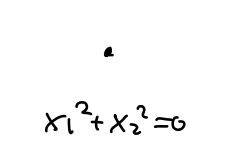• $$x_1^2-x_2^2=0$$, yielding a split node and rational tangent directions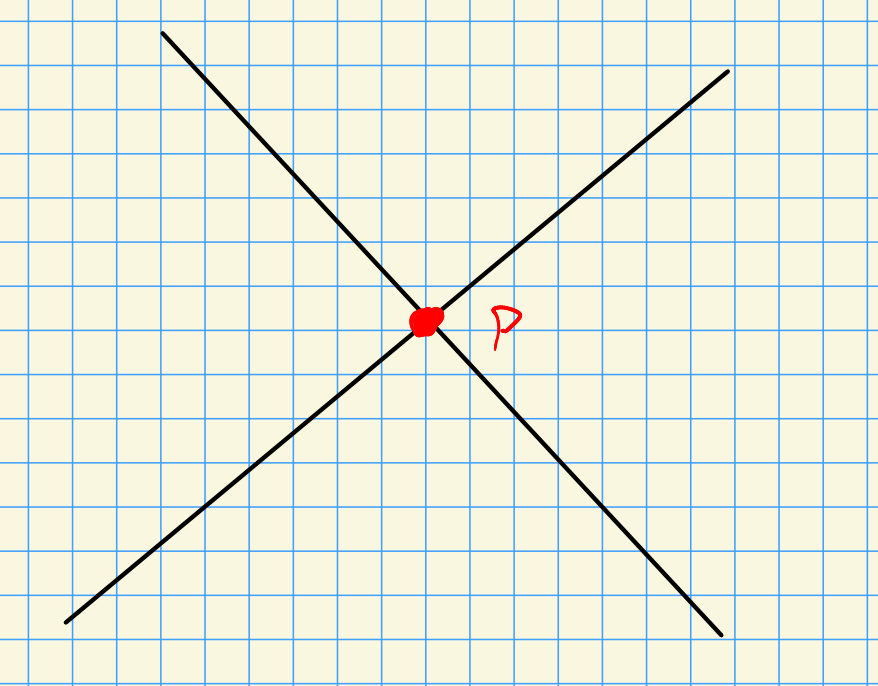Let $$p$$ be a node with \begin{align*} \widehat{{\mathcal{O}}_{X, p}} { { \, \xrightarrow{\sim}\, }}{ L { \left[\left[ { { {x}_1, {x}_2, \cdots, {x}_{n}} } \right] \right] } \over \sum a_i x_i^2 }, && L \mathrel{\vcenter{:}}=\kappa(p) .\end{align*} The type $$p$$ is defined as \begin{align*} {\operatorname{type}}(p) \mathrel{\vcenter{:}}=\operatorname{Tr}_{L_{/ {k}} } \left\langle{2^n \prod_{i=1}^n a_i}\right\rangle \in {\operatorname{GW}}(k) .\end{align*}

The $${\mathbb{A}}^1{\hbox{-}}$$Milnor number is defined as \begin{align*} M_p \mathrel{\vcenter{:}}=\deg_p^{{\mathbb{A}}_1} \operatorname{grad}f = \sum_{p\in N} {\operatorname{type}}(p) ,\end{align*} $$N$$ is the set of nodes of $$f$$ in a family for a generic $$\left\{{{ {a}_1, {a}_2, \cdots, {a}_{n}} }\right\}$$.

Note: the second equality is due to Kass-Wickelgren.

Let $$f(x,y)\mathrel{\vcenter{:}}= x^3-y^2$$ with $$\operatorname{ch}k\neq 2,3$$, then

• $$p=(0, 0) \in \left\{{f=0}\right\}$$,
• $$\nabla f = (3x^2, -2y)$$, and \begin{align*} \deg^{{\mathbb{A}}^1} \nabla f &= \deg^{{\mathbb{A}}^1} (x\mapsto 3x^2 ) \deg^{{\mathbb{A}}^1} (y\mapsto -2y) \\ &= { \begin{bmatrix} {0} & {1/3} \\ {1/3} & {0} \end{bmatrix} } \left\langle{-2}\right\rangle \\ &= \left\langle{1}\right\rangle + \left\langle{-1}\right\rangle \\ &= q_{{\operatorname{hyp}}} ,\end{align*} which has rank 2 and thus $$M = 2$$. This yields a cusp.

The family $$y^2 = x^3 + ax + t$$ for $$a\neq 0$$ yields a family: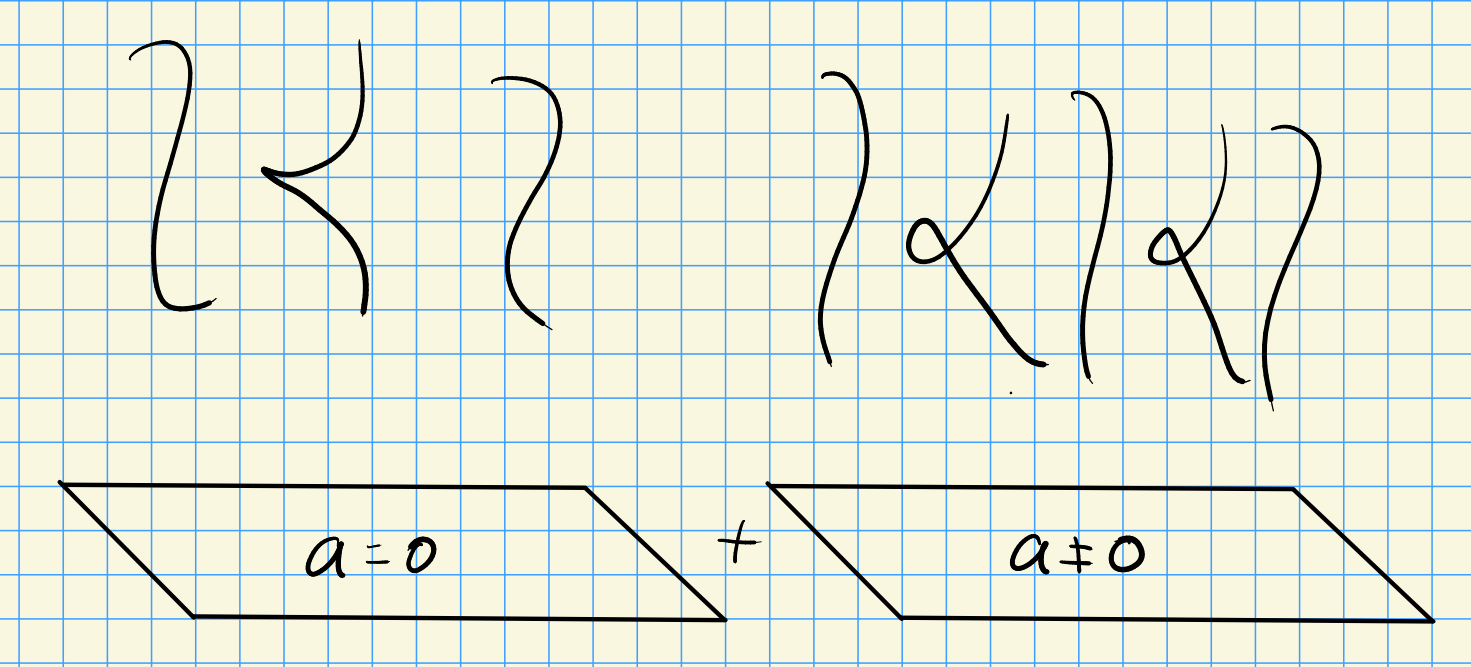• In the first term, the cusp bifurcates into 2 nodes, yields $$\operatorname{rank}M^{{\mathbb{A}}^1}(C) = M(C)$$ for $$C$$ the cusp curve
• In the second, there are singular fibers when $$x^3+ax+t$$ has double roots, which happens when the discriminant is zero, so this occurs iff $$-4a^3 -27b^2 =0$$.

This yields a bifurcation into two nodes, and for example,

• Over $${\mathbb{F}}_5$$: $$\left\langle{1}\right\rangle = \left\langle{-1}\right\rangle$$, so this can not bifurcate into 1 split and 1 non-split rational node.
• Over $${\mathbb{F}}_7$$: $$\left\langle{1}\right\rangle \neq \left\langle{-1}\right\rangle$$, so this can not bifurcate into 2 split or 2 non-split nodes.

The classical Milnor number appears in conductor formulas and are related to the Euler characteristic $$\chi$$ of the Milnor fiber. M. Levine-Lehalleur-Srinivas and R. Azouri have subtle quadratic enrichments to $${\operatorname{GW}}(k)$$.

## 2.3$${\mathbb{A}}^1{\hbox{-}}$$Euler characteristic $$\chi^{{\mathbb{A}}^1}$$

For $$X\in {\mathsf{sm}}{\mathsf{Var}}^{\mathop{\mathrm{proj}}}_{/ {k}}$$, we saw last time that a vector bundle $$V\to X$$ is relatively oriented by $$(L, \phi)$$ where $$L\to X$$ is a line bundle and \begin{align*} \phi: L{ {}^{ \scriptstyle\otimes_{k}^{2} } } \xrightarrow{\sim} \mathop{\mathrm{Hom}}(\operatorname{det}{\mathsf{T}}X, \operatorname{det}V) .\end{align*}

The tangent bundle $${\mathsf{T}}X$$ has a canonical relative orientation since \begin{align*} \mathop{\mathrm{Hom}}(\operatorname{det}{\mathsf{T}}X, \operatorname{det}{\mathsf{T}}X) \xrightarrow{\sim} {\mathcal{O}}\xrightarrow{\sim} {\mathcal{O}}{ {}^{ \scriptstyle\otimes_{}^{2} } } \end{align*} where $${\mathcal{O}}$$ is a trivial bundle of rank 1. It follows that we may define the Euler number of tangent bundle: \begin{align*} \chi^{{\mathbb{A}}^1}(X) \mathrel{\vcenter{:}}= n({\mathsf{T}}X) \in {\operatorname{GW}}(k) ,\end{align*} where $$n$$ is the Euler number.

$$\chi^{{\mathbb{A}}^1}(X)$$ is equal to the categorical Euler characteristic. The definition is omitted here, see Levine’s “Enumerative geometry with quadratic forms”.

Let $$X \subseteq {\mathbb{P}}^{n+1}$$ for $$n$$ even, written $$X \mathrel{\vcenter{:}}=\left\{{F=0}\right\}$$ for $$f\in {\mathbb{R}}[x_0, \cdots, x_n]_{e}$$ homogeneous of degree $$e$$. Take \begin{align*} \omega^{{\mathrm{EKL}}}: Q{ {}^{ \scriptstyle\otimes_{}^{2} } }\to k && Q \mathrel{\vcenter{:}}={k[x_0,\cdots, x_n] \over \left\langle{ {\frac{\partial f}{\partial x_0}\,}, \cdots, {\frac{\partial f}{\partial x_n}\,} }\right\rangle } .\end{align*}

Define $$B_{\operatorname{Jac}}$$ to be the restriction of $$\omega^{\mathrm{EKL}}$$ to $$\bigoplus_{q=0}^n Q_{(q+1)e-n-2}$$, then \begin{align*} \chi^{{\mathbb{A}}^1}(X) = \left\langle{e}\right\rangle + \left\langle{-e}\right\rangle B_{\operatorname{Jac}} + {n\over 2}q_{{\operatorname{hyp}}} .\end{align*}

Take the Clebsch surface \begin{align*} C \mathrel{\vcenter{:}}=\left\{{ {\left[ {{ {x}_1, {x}_2, \cdots, {x}_{3}}} \right]} {~\mathrel{\Big|}~}\sum x_i^3 = \qty{\sum x_i}^3 }\right\} \subseteq {\mathbb{P}}^3_{/ {{\mathbb{Q}}}} .\end{align*} Then $$\chi(C) = 9$$, and \begin{align*} \chi^{{\mathbb{A}}^1}(C) = 2q_{{\operatorname{hyp}}} + \left\langle{-10}\right\rangle + \left\langle{-6}\right\rangle + \left\langle{-21}\right\rangle + \left\langle{-14}\right\rangle + \left\langle{-2}\right\rangle ,\end{align*} which can be computed with Macaulay2!

## 2.4 Cohomology and the Euler Class

Note that we have a stable $${\mathbb{A}}^1{\hbox{-}}$$homotopy category $${\mathsf{SH}}(k)$$, so we can take cohomology theories on $$X\in {\mathsf{sm}}{\mathsf{Sch}}_{/ {k}}$$:

• $$H_{ \mathrm{mot}}$$ or $$H{\mathbb{Z}}$$: motivic cohomology
• $$\tilde H_{ \mathrm{mot}}$$ or $$H\tilde {\mathbb{Z}}$$: extended motivic cohomology
• $${\mathsf{K}}$$: $${\mathsf{K}}{\hbox{-}}$$theory
• $${\operatorname{KO}}$$: Hermitian $${\mathsf{K}}{\hbox{-}}$$theory

It turns out that \begin{align*} H^n(X) = \pi_{-n}\mathop{\mathrm{Hom}}_{{\mathsf{SH}}(k)}(X, H) = [X, {\Sigma}^n H] ,\end{align*} and it’s useful to allow twisting the second term by shifts.

Letting $$V\to X$$ be a vector bundle, we can define the twisted suspension \begin{align*} H^V(X) \mathrel{\vcenter{:}}=[X, \mathop{\mathrm{Th}}(V) \wedge H] && \text{where } \mathop{\mathrm{Th}}(V) \mathrel{\vcenter{:}}={V {}^{ \vee }\over V {}^{ \vee }\setminus\left\{{0}\right\}} = {{\mathbb{P}}(V {}^{ \vee }\oplus {\mathcal{O}}) \over {\mathbb{P}}(V {}^{ \vee })} \end{align*} where taking the dual is a condition needed for $$K{\hbox{-}}$$theory. This reduces to a usual suspension for the trivial bundle.

For $$V\mathrel{\vcenter{:}}=({\mathcal{O}}_X){ {}^{ \scriptscriptstyle\oplus^{n} } }$$ the trivial rank $$n$$ bundle on $$X$$, we have $$H^V = H^n$$.

\begin{align*} H{\mathbb{Z}}^n(X) \cong H_{ \mathrm{mot}} ^{2n}(X; {\mathbb{Z}}(n)) \cong H^{2n, n}_{ \mathrm{mot}} (X) \cong {\operatorname{CH}}^n(X) ,\end{align*} the Chow group of codimension $$n$$ cycles modulo rational equivalence. These are the geometric gradings.

$$\tilde{H{\mathbb{Z}}}^n(X) \cong \tilde{{\operatorname{CH}}}^n(X)$$ the Chow-Witt group, also called the oriented Chow group. Using the Gersten resolution, these can be expressed as formal sums of codimension $$n$$ subvarieties with coefficients in $${\operatorname{GW}}(k(Z))$$, where $$k(Z)$$ are the rational functions of $$Z$$, subject to some conditions, modulo equivalence.

$${\mathsf{K}}^0(X)$$ is the group completion of vector bundles on $$X$$, and $${\operatorname{KO}}^0(X)$$ additionally remembers the data of a nondegenerate symmetric bilinear form. These are “representable” in a sense because they are related to Thom spectra.

Let $$Z\hookrightarrow X$$ be a closed subscheme, then define the cohomology with support \begin{align*} H_Z^V(X) \mathrel{\vcenter{:}}= { \left[ { { X \over X\setminus Z}, \mathop{\mathrm{Th}}(V) \wedge H} \right] } _{{\mathsf{SH}}(k)} .\end{align*}

For $$H$$ a cohomology theory and $${\mathbb{S}}|to H$$ a ring? and a vector bundle $$V\to X$$ with a section $$f$$ (for example, the zero section), then the Euler class \begin{align*} e^H(V, f) \in H_{\left\{{f=0}\right\}}^{V {}^{ \vee }}(X) \end{align*} is the class of the map \begin{align*} {X\over X\setminus\left\{{f=0}\right\}} \xrightarrow{f} {V\over V\setminus\left\{{0}\right\}} \wedge H .\end{align*}

For $$f:X\to S$$ is a local closed immersion ($$\mathrm{lci}$$) if it locally factors as $$U \xrightarrow{i} P \xrightarrow{p} S$$ with $$p$$ smooth and $$i$$ a closed immersion determined by a Koszul regular sequence (so modding out doesn’t yield a zero divisor, and the higher cohomology of the Koszul complex is zero).

Some properties:

• This has a well-behaved cotangent complex $$L_f$$.

• For a regular embedding, $$L_i \simeq N_u {}^{ \vee }P$$ is conormal bundle.4

• $$L_p \simeq\Omega_{P/S} \simeq{\mathsf{T}}_p {}^{ \vee }$$.

• $$L_{p_i}$$ is determined by $$i^* L_p\to L_{p_i} \to L_i$$,

• There is a coherent Serre duality related to $$L_f$$.

There is also a good notion of pushforward: let $$p:X\to S$$ be proper5 and $$\mathrm{lci}$$.

Then there is a Becker-Gottlieb transfer \begin{align*} \operatorname{Tr}^{\text{BG}}: {\Sigma}_+^\infty S\to \mathop{\mathrm{Th}}(L_p) ,\end{align*} and the cartoon is the following: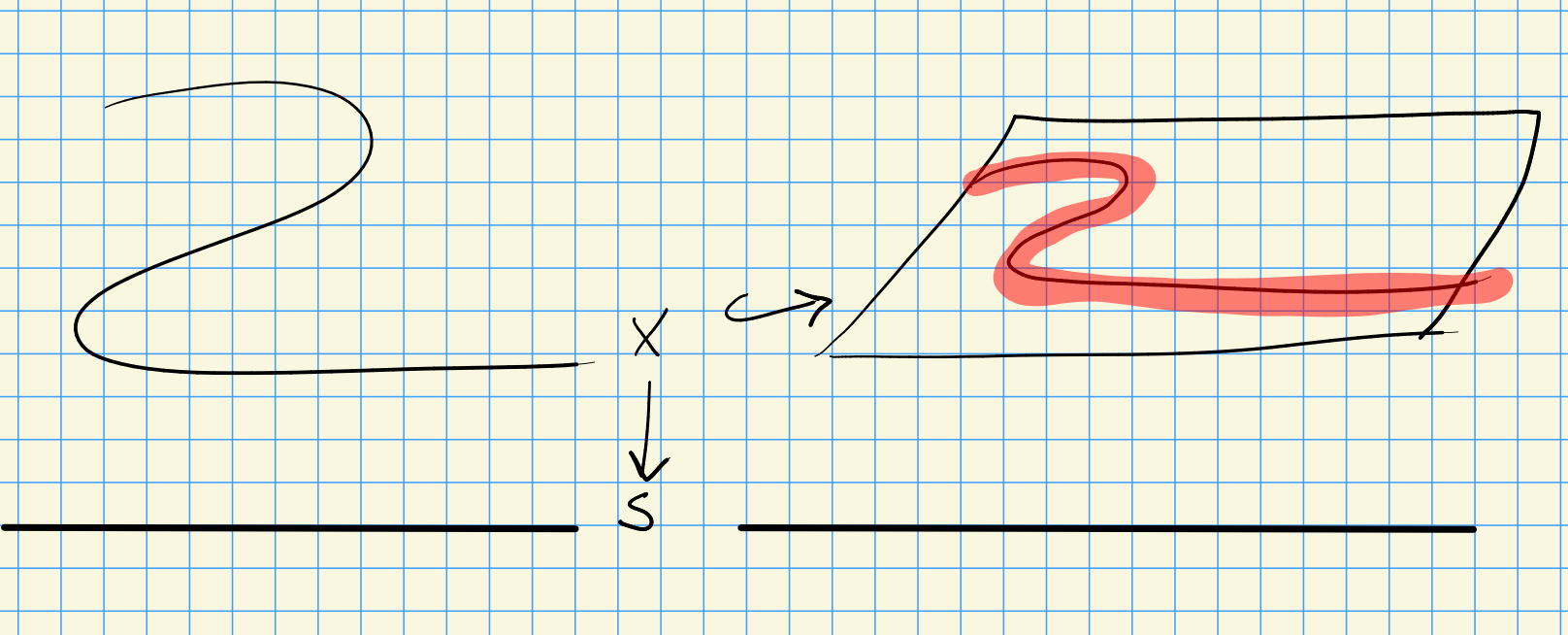Here we embed $$X\to S$$ into the trivial bundle over $$S$$ and take a neighborhood. Letting $$B$$ be the trivial bundle over $$S$$, then $$\mathop{\mathrm{Th}}(B) \cong \Sigma_+^\infty S$$ and collapsing fiberwise quotients by the complement of the neighborhood: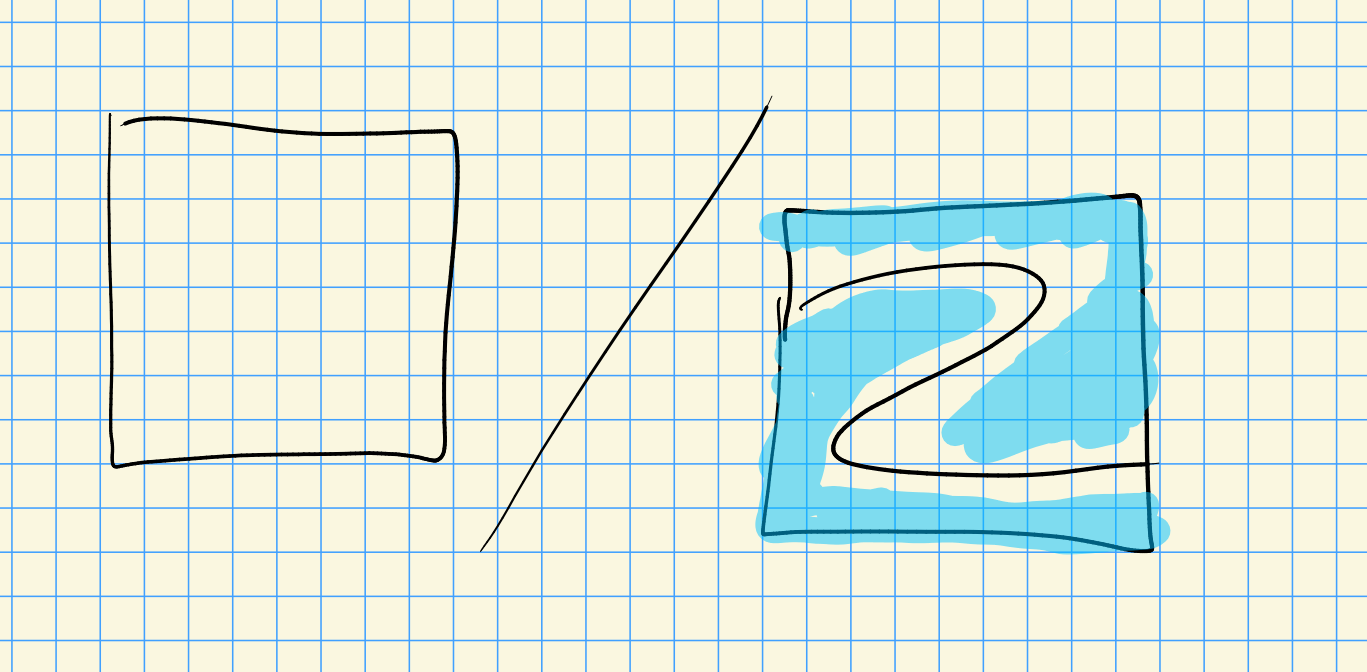This yields $$p_*: H^{L_p}(X) \to H^0(S)$$.

## 2.5 Oriented Cohomology Theories and Euler Numbers

• $$H$$ is $$\operatorname{GL}{\hbox{-}}$$oriented if $$H_Z^n(X) \xrightarrow{\sim} H_Z^V(X)$$ with $$n\mathrel{\vcenter{:}}=\operatorname{rank}V$$.

An example is $$H{\mathbb{Z}}, {\mathsf{K}}$$, but non examples are $${H\tilde {\mathbb{Z}}}, {\operatorname{KO}}$$.

• $$H$$ is $${\operatorname{SL}}{\hbox{-}}$$oriented if

• $$H_Z^V(X) \xrightarrow{\sim} H_Z^{V'}(X)$$,
• $$\operatorname{rank}V = \operatorname{rank}V'$$ and
• $$\operatorname{det}V \xrightarrow{\sim} \operatorname{det}V' \otimes L{ {}^{ \scriptstyle\otimes_{}^{2} } }$$ for $$L\to X$$ a line bundle.

An example is $$H\tilde{{\mathbb{Z}}}, {\operatorname{KO}}$$.

For $$V\to X$$ a relatively oriented vector bundle on $$X \xrightarrow{p} k$$ with $$p$$ smooth and proper, and $$H$$ an $${\operatorname{SL}}_c{\hbox{-}}$$oriented cohomology theory. Then \begin{align*} H^{V {}^{ \vee }}(X) \xrightarrow{\sim} H^{{\mathsf{T}} {}^{ \vee }X}(X) .\end{align*} Letting $$f$$ be any section of $$V$$, e.g. the zero section, thenLetting $$f: X\to \mathop{\mathrm{Th}}(V) \wedge H$$, any two sections $$f_1, f_2$$ of $$V$$ are connected by copies of $${\mathbb{A}}^1$$ in $$H^0(V)$$, so \begin{align*} e^H(V) \mathrel{\vcenter{:}}= z(e^H(V, f_1)) = z(e^H(V, f_2)) .\end{align*}

The Euler number $$n^H(V)$$ of $$V$$ in $$H^0(S)$$ is \begin{align*} n^H(V) \mathrel{\vcenter{:}}= p_* e^H(V) .\end{align*}

This agrees with \begin{align*} n(V, f) = \sum_{x\in \left\{{f= 0 }\right\} \subseteq X} \deg_x f, && \deg_x f \in {\operatorname{GW}}(k) ,\end{align*} for $$H = H\tilde{{\mathbb{Z}}}, {\operatorname{KO}}$$ over $$S\mathrel{\vcenter{:}}=\operatorname{Spec}k$$. Moreover, $$n(V, f)$$ is independent of the choice of section.

## 2.6 Arithmetic count of lines on a smooth cubic surface (joint with Jesse Kass)

A cubic surface is given by $$X \subseteq {\mathbb{P}}^3$$ where $$X = \left\{{f=0}\right\}$$ with $$f\in k[w,x,y,z]_3$$ homogeneous of degree 3.

Any smooth cubic surface over $${\mathbb{C}}$$ contains exactly 27 lines.

Consider the Fermat cubic: \begin{align*} f(x,y,z,w) = x^2 + y^2 + z^2 + w^2 .\end{align*} The lines are given by \begin{align*} \left\{{ {\left[ {S, -S, T, -T} \right]} {~\mathrel{\Big|}~}[S, T] \in {\mathbb{P}}^1({\mathbb{C}}) \subseteq X }\right\} .\end{align*} For $$\omega^3,\lambda^3 = 1$$, we can take \begin{align*} \left\{{{\left[ {S, \lambda S, T, \omega T} \right]} {~\mathrel{\Big|}~}[S, T]\in {\mathbb{P}}^1({\mathbb{C}}) }\right\} .\end{align*} Permuting the variables in $${4\choose 2}/2 =3$$ ways, then there are $$3\cdot 3 \cdot 3 = 27$$ total lines.

Let $${\operatorname{Gr}}(1, 3)$$ be the Grassmannian parameterizing $$W \subseteq {\mathbb{C}}^4$$ with $$\dim W=2$$, or equivalently copies of $${\mathbb{P}}^1$$ in $${\mathbb{P}}^3$$. Take the tautological $$S\to {\operatorname{Gr}}(1, 3)$$ whose fiber over $$[{\mathbb{P}}W]$$ is $$W$$ itself. Then $$\operatorname{Sym}^3 S {}^{ \vee }_{[{\mathbb{P}}W]} = \operatorname{Sym}^3(W {}^{ \vee })$$ are cubic polynomials in $$W$$, and $$f$$ determines a section $$\sigma_f$$ of $$\operatorname{Sym}^3 S {}^{ \vee }$$ given by \begin{align*} \sigma_f([{\mathbb{P}}W) = { \left.{{f}} \right|_{{W}} } ,\end{align*} which is zero iff the line $${\mathbb{P}}W$$ is contained in $$X$$. So we’ve reduced to counting zeros of a section: \begin{align*} n(V) = \sum_{\text{lines } L \subseteq X} \deg_L \sigma_f .\end{align*}

For a smooth cubic surface, all zeroes of $$\sigma_f$$ have multiplicity one.

Over $${\mathbb{C}}$$, the classical differential topological degree is 1, so $$n(V)$$ is the number of lines, and $$n(V) = 27$$.

Consider now the situation over $${\mathbb{R}}$$:

• Schläfli (1861): Over $${\mathbb{R}}$$, there can only be $$3, 7, 15$$, or $$27$$ lines.
• Segre (1942): These lines break into two classes: hyperbolic, or elliptic.

For $$L \subseteq X$$ a real line, $$L$$ gives an involution $$I:L\to L$$ where $$I(p)$$ is defined by consider $$T_p X$$, which contains a line $$L$$ along with a curve $$C$$ of degree 2 since the total degree is 3: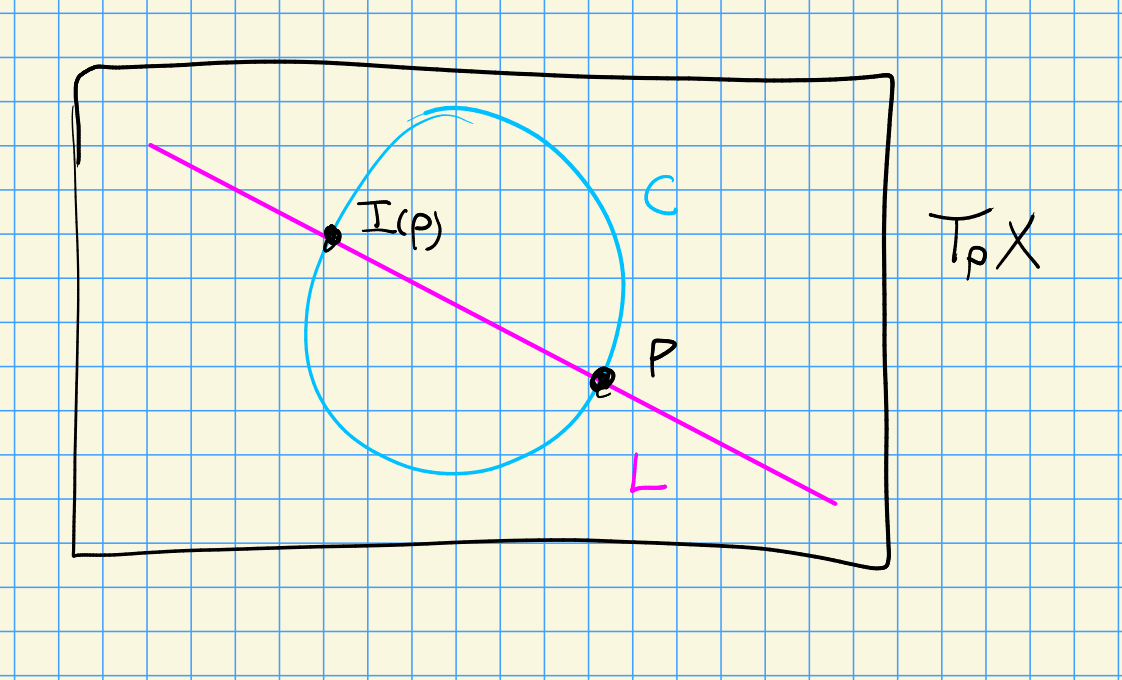In particular, there is another point in the intersection of $$L \cap C \cap X$$, so $$I(p)$$ is defined as that point where $$T_{I(p)}X = T_pX$$. If $$I$$ yields a $${\mathbb{C}}{\hbox{-}}$$conjugate pair, say $$L$$ is elliptic, and otherwise if $$I$$ yields two $${\mathbb{R}}{\hbox{-}}$$point say $$L$$ is hyperbolic. Taking a path $$p\to I(p)$$, if the tangent space undergoes a full twist, this is the elliptic situation.

The number of hyperbolic lines minus the number of elliptic lines is exactly 3.

What about other fields, like $$k = {\mathbb{F}}_p, { {\mathbb{Q}}_p }, {\mathbb{Q}}$$?

The above proof works in $${\mathbb{A}}^1{\hbox{-}}$$homotopy theory. Letting $$X \subseteq X \subseteq {\mathbb{P}}^3_{/ {k}}$$ a cubic surface.

The type of a line $$L$$ is an element \begin{align*} \left\langle{D}\right\rangle \in {\operatorname{GW}}(k(L)), && D \in k(L)^{\times}/ (k(L)^{\times}){ {}^{ \scriptscriptstyle\times^{2} } } \end{align*} such that the fixed points $$\mathrm{Fix} (I)$$ form a conjugate pair of points defined over $$k(L) { \left[ {\sqrt D} \right] }$$.

let $$k\in \mathsf{Field}^{\operatorname{ch}\neq 2}$$ and $$X \subseteq {\mathbb{P}}^3_{/ {k}}$$, then \begin{align*} \sum_{\substack{\text{lines} \\ L \subseteq k(X) }} \operatorname{Tr}_{k(L) / k} {\operatorname{type}}(D) = 15\left\langle{1}\right\rangle + 12\left\langle{-1}\right\rangle \in {\operatorname{GW}}(k) .\end{align*}

# 3 Bibliography

1. The dualizing sheaf is locally free↩︎

2. Even better, there is a distinguished isomorphism coming from a distinguished socle element (Scheja-Storch).↩︎

3. Her $${\mathcal{O}}_{X, p}$$ is the stalk of the structure sheaf at $$p$$, which is a local ring with a unique maximal ideal $${\mathfrak{m}}_p$$, and the LHS is completion at that ideal, so \begin{align*} \widehat{{\mathcal{O}}_{X, p}} \mathrel{\vcenter{:}}=\qty{{\mathcal{O}}_{X, p}}{ {}_{ \widehat{{\mathfrak{m}}_p} } } .\end{align*} ↩︎

4. I.e. the dual of the normal bundle.↩︎

5. Here proper means that the preimage of a compact set is compact.↩︎